# Overview

As a student of data science, I recently learned how to model variable interactions using Ordinary Least Squares (OLS) linear regression. It struck me as strange that the common advice to avoid the Dummy Variable Trap when analyzing categorical variables is to simply drop the first column based on the alpha-numeric category labels.

My intuition was that it must matter to some degree which column we choose to drop. And if it does matter, dropping a column because its label comes first seems very arbitrary and not especially scientific.

I found that while there are plenty of web resources describing why we drop a column in this scenario, few attempted to address the question of which to choose. So, to satisfy my curiosity and strengthen my understanding of OLS linear regression, I created some experimental data and tested the results of dropping different columns. My goal was to determine:

For those of you who want to get right to the point, my analysis revealed:

While the column dropped does not appear to affect an OLS linear regression model’s performance, it can have a significant impact on the interpretability of the model’s coefficients.

Data scientists preparing an Ordinary Least Squares multiple linear regression model should carefully consider which columns they drop from each category if their goals for analysis include:

Arbitrarily dropping the first column without considering what it represents can make it difficult to interpret the model coefficients not only with accuracy, but also in a way that will be intuitive for non-technical stakeholders.

Instead of relying on the convention of dropping the first column, data scientists should consider dropping columns that represent logical baseline reference points, from which the model will assume all included predictor variables to deviate. In some cases, a minimum value may be appropriate, in other cases an average value may be appropriate, and in still others the appropriate reference category may depend entirely on the goal of the analysis.

Below, I first provide some background, then outline the steps I took to come to these conclusions. The full details of my approach and analysis can be found in my github repo.

# Background

## What is one-hot encoding?

When preparing data for a linear regression model, it is necessary to dummy, or one-hot encode (OHE), categorical variables into separate columns to represent the non-numerical values as numbers.

A categorical variable which represents gender, for instance, will be transformed from a single column into multiple one-hot encoded columns, so named since a value of 1 represents membership in that category with the rest of the category columns being filled with zeros.

## Why drop any columns at all?

When learning about linear regression, students of data science are taught to drop one column from each categorical variable group to act as the reference category, and to avoid the “Dummy Variable Trap:” perfect multicollinearity between the predictors.

In our example, we could drop Female, Male, or NonBinary and leave the other two columns in our model.

As this article illustrates it would not be possible to solve the OLS problem at all using linear algebra if we didn’t drop a column, since we would have a singular matrix. Although both scikit-learn and statsmodels libraries use a different algorithm to solve OLS, and so technically can generate models if we don’t drop a column from each category, it’s still considered best practice to do so. In my experiment, I included models with no columns dropped to illustrate the strange results.

If the dataset contains multiple categorical variables, one column should be dropped from each group of resulting OHE columns. Throughout this article, I will discuss dropping ‘a column,’ which should be understood to mean one column from each categorical variable’s group.

Note that it’s necessary to drop one column from an OLS Linear Regression model, but may not be necessary (or even ill-advised) with other model types. The conclusions reached here are applicable to OLS linear regression models.

## Arbitrarily dropping “first”

Python libraries such as Pandas and sckikit-learn have parameters built in to their one-hot-encoding methods which allow us to drop a column from each categorical group. A common approach is to drop first, meaning drop whichever column represents the category value name that comes first alpha-numerically in the set. In our gender example, the first column would be Female since F comes before M and N.

In fact, dropping first seems to be such a common convention that both Pandas and scikit-learn have handy constants you can use to do this so you don’t have to figure out what the first category is.

But is it really the best way to choose a variable to drop in all situations? In the following sections, I’ll walk through how I created data to test this, and concluded that dropping the first column is not always the best option.

# Creating the test data set

I created a test data set of n=20,000 based on home characteristics and sales, where my OLS linear regression model would be trying to predict a home’s eventual sale price.

First, I generated the independent (predictor) variables: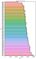Average Price per Zip Code Category, ranked from lowest to highest

With my Sq Ft values randomly generated, and houses assigned to categories as described above, I applied the multipliers to generate Price. I used a starting baseline of \$100,000 (which became the expected y-intercept), and applied categorical multipliers to a constant of \$50,000.

I now had a target variable, Price, which had been generated based on known coefficients of each predictor variable.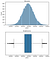Histogram and Boxplot for target variable Price, which was generated from predictor coefficients

I noted the expected coefficients for each predictor category in original units, as well as the coefficient in standardized units (standard deviations) for the continuous variable, Square Feet. This allowed me to compare the results from each model against expected coefficients.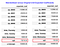Standardized expected coefficients on the left, original units on the right. They are the same for categorical variables, and only different for Sq Ft, since categorical variables do not need to be standardized further.

# Modeling

My primary question was whether the category column dropped from the model (i.e. used as the model’s reference point) would affect the model’s results. I wanted to test dropping the first column, since it’s the common convention, and as the alternative I wanted to drop a column representing the average category: the category in which mean home Price most closely matched the population mean.

There were three different types of results I was interested in:

So I needed at minimum:

I decided to also run models where no categorical columns were dropped just to see what happened. And finally, I varied whether I included the DOTW variable in the model or not, where including it should allow the model to predict 100% of the target’s variability, and excluding it would introduce some errors and should be slightly more realistic.

Ultimately, I ended up iterating through 12 models with these different parameters, and then reviewed the results.

# Model Interpretation and Conclusions

To evaluate performance, I used a train-test split and generated the R-squared, Residual Sum of Squares (RSS), and Root Mean Squared Error (RMSE) for both train and test.

To evaluate interpretability, I compared the test model coefficients to expected, reviewed how test models differed from each other, and considered how accurate my natural conclusions would be.

## Performance

Being able to predict the target given the independent variables is one of the key measures of success for a linear regression model.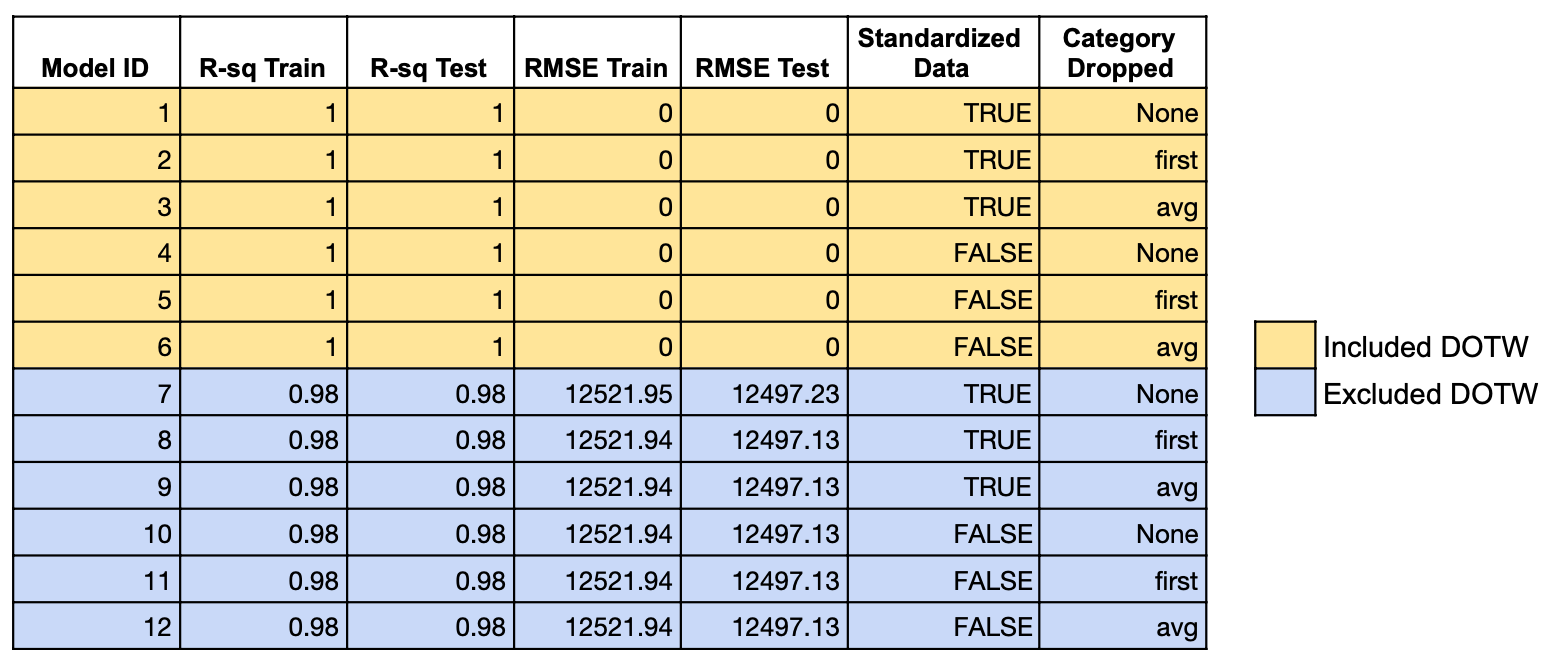Performance statistics R-squared, and RMSE for each model. We can see that when the confounding variable DOTW was included, all versions of the models were perfect. When we didn’t include DOTW, we see some errors introduced as we would expect, but varying the category dropped or whether the data was standardized didn’t affect the results.

In the first 6 models, I included the ‘day of the week’ variable, and in the last 6 I left it out, so it would act as a confounding variable.

Although other aspects of how the data was processed were varied, such as whether the data was standardized and which category was dropped, these factors do not have an appreciable affect on R-Squared, Root Mean Squared Errors, or Residual Sum of Squares (not shown above for brevity). In other words, the first 6 models look the same, and the last 6 models look the same.

The models which were run without the ‘day of the week’ variable were not perfect, which makes intuitive sense. But there doesn’t appear to be any difference between these models where I excluded DOTW; they have the same R-squared and RMSE.

I concluded that varying which category column is dropped from the model does NOT affect the model’s performance.

## Interpretability — Accurately Ranking Standardized Coefficients

Another way we might want to be able to use our model is to rank the standardized coefficients to compare the magnitude of their effects on the target, or their importance. The goal would be to determine which variables increase or decrease the target to a greater degree. It’s important to standardize continuous variables so their coefficients are in standard deviation units that can be compared.

These insights, combined with domain knowledge, could be instrumental for business stakeholders to decide on an appropriate action plan. If their goal is to tweak predictors to affect the target, knowing which predictors have the largest impact is key.

To measure how accurate the ranked coefficients of our models would be, consider the heatmaps below. Although each model’s heatmap uses its own scale for the color gradient, we would expect the general gradient order of the test models to match the gradient order of the expected model.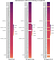Comparing standardized coefficients of test models to expected. Does not include y-intercept.

In both models, Zip Codes look to follow the expected order pretty well. But in the Dropped First mode, the Condition categories and Square Feet don’t look right; their colors pop out of the gradient because they have much lower standardized coefficients than we expected.

In the Dropped Average model, all of the predictor variables look pretty close.

Note that the y-intercepts of both test models are quite a bit different than expected. In fact, the Dropped Average model’s y-intercept is quite different. But it’s unlikely we would be concerned with ranking the y-intercept accurately; we would probably be most concerned with ranking the actual predictor variable coefficients.

Let’s remove the Zip Code categories to zoom in on the others.

If we take them out of the context of Zip Codes, it does look like the order of Condition categories matches expected for both models. In other words, Excellent Condition adds more than Above Average, which are above Average, etc.

However, Square Feet should add about \$55k more to Price than Excellent Condition. We see that this difference is pretty accurate in the Dropped Average model, but in the Dropped First model, Excellent Condition is ranked above Square Feet.

If I were to use the standardized Dropped Average model to estimate which variables were more important to increasing price, I’d be pretty accurate.

But if I were to use the standardized Dropped First model to estimate this, I’d assume that Zip Codes were by and large the most important, followed by Condition Excellent, and then Square Feet. This would not be very accurate.

## Accurately AND Intuitively Interpreting Original Unit Coefficients

We also might be interested in using the coefficients in original units, from the model where we did not standardize the data, to understand how the target variable changes per unit of each predictor variable.

For instance, we would want to be able to use the coefficient associated with Square Feet to say “For each sq ft added, the price goes up by an estimated \$100.”

I’ve created similar heatmaps to review original unit coefficient accuracy. Note that in this visualization, the color scales of both test models were forced to match the expected model, so we can compare the values by comparing the shades directly. Here, we care about how accurate the coefficients are, as opposed to with standardized coefficients, where we cared more about the ranking order.

We can see that the Dropped Average model’s coefficients are very close to expected values. Its colors are very similar to expected, and in the right order.

However, in the Dropped First model, the y-intercept was very low, which caused most of the other coefficients to be too high to make up for it.

We can see that the Square Feet coefficient is pretty close, but Zip Code values are quite high, and it’s difficult to determine where Condition falls. Let’s remove Zip Codes to zoom in on the other variables.

We’ll take some time to interpret the differences we see here, as the fact that the Dropped First model’s unit coefficients do not exactly match expected do not necessarily mean they are inherently wrong or not useful.

First, let’s notice that both test models have very accurate unit coefficients for Square Feet, our only continuous variable.

We can see clearly that the Dropped Average model has unit coefficients for Condition variables that very closely match expected, while the Dropped First model coefficients do not. Practically, what does this mean?

It’s important to put these unit coefficients in the context of each model’s baseline, or reference point. The coefficients must be interpreted with respect to this baseline.

For our continuous variable, Square Feet, the reference point was 0, and this would be the case for any continuous variables we included.

But for our categorical variables, the reference point in the baseline became whichever category column we dropped from the model. So we can say that:

The differences in unit coefficients between the two test models is due to the models having different reference points for their categorical values.

Now it makes more sense why the coefficient for Square Feet is the same for both models, but the categorical coefficients differ: Square Feet assumed a baseline of 0 in both models.

The next logical question to ask is: Is one baseline inherently better than the other? Since we saw that model performance doesn’t change, it’s not immediately clear. To answer this question, let’s consider how we would interpret the Condition unit coefficients for the Dropped First model, assuming we didn’t know what was expected.

In the Dropped First model, we dropped the Poor Condition column. So our baseline assumes a reference point of having the worst possible condition. We would interpret the coefficients like:

In the Dropped First model, we’re interpreting each Condition level as adding to the baseline, even Below Average. Whereas with the Dropped Average model, the baseline assumes a condition of Average, so we would interpret the coefficients like:

The steps between the coefficient values are the same in both models, but the positive/negative aspect is different.

One is not objectively better than the other, but:

If the goal of analysis is to provide actual coefficient values to non-technical stakeholders, dropping the average category for Condition yields the most intuitive coefficients that are more likely to make sense to stakeholders.

Imagine the difficulty of explaining that although we have a positive number for the impact of Below Average Condition, this is within the context of a baseline house that actually has a negative price… it really doesn’t make much sense. It’s not inherently wrong, but without the right framing, people may come to misleading conclusions.

# Summary

Although the category you choose to drop won’t affect the model’s performance, it can have a significant impact on the interpretability of the model.

If you plan to use the coefficients in your model to make accurate inferences about:

then you should carefully consider which categorical values you drop from your model.

A linear regression model’s coefficients are interpreted in the context of a baseline model. For continuous variables, the baseline uses a reference point of 0. But for categorical variables, whichever column is dropped becomes the reference point, which has a significant impact on how coefficients are interpreted.

It’s important for data scientists to consider which columns represent the most intuitive reference points for each category, and drop those. Simply dropping the first column is arbitrary: the first column will not necessarily represent the minimum, and also the minimum does not necessarily always make a good reference point.

A thoughtful choice of which column to drop will yield coefficients that are much easier to interpret accurately.

# How should you choose which column to drop?

Instead of assuming that the first or minimum category value is the most appropriate, consider which category represents the most intuitive reference point for your stakeholders, or to help answer the questions at the root of your analysis.

For example, if you were modeling predictors of salary you might have a categorical predictor for level of schooling with the following values:

If you relied on the alpha-numeric first column, then Associate’s degree would be your reference point. This could make sense if your stakeholders would be interested in how much people with less than an Associate’s degree earn compared to people with higher than an Associate’s degree.

But it could also be reasonable to use High School or GED as the reference point, if you felt that was a more accurate representation of the population’s average education attainment. You could even figure out which of these categories seems to have a mean or median salary that most closely matches the mean or median of the population, to have a statistical reason to choose a particular category as the reference point.

In this experiment, I dropped the categories that represented the average. That made sense because of what my categories represented. To select the appropriate column for a given scenario, data scientists will need to consider which column represents an intuitive reference point for their stakeholders, in the context of which, positive and negative coefficients will have relevant meaning.

And of course, if your primary goal is prediction, you don’t have to worry about which category value you drop!

I hope others find this useful and informative. As I’m still a student of data science, I’d welcome any thoughts on my testing approach or applicability of my conclusions.

## More from Jessica Miles

Student of Data Science